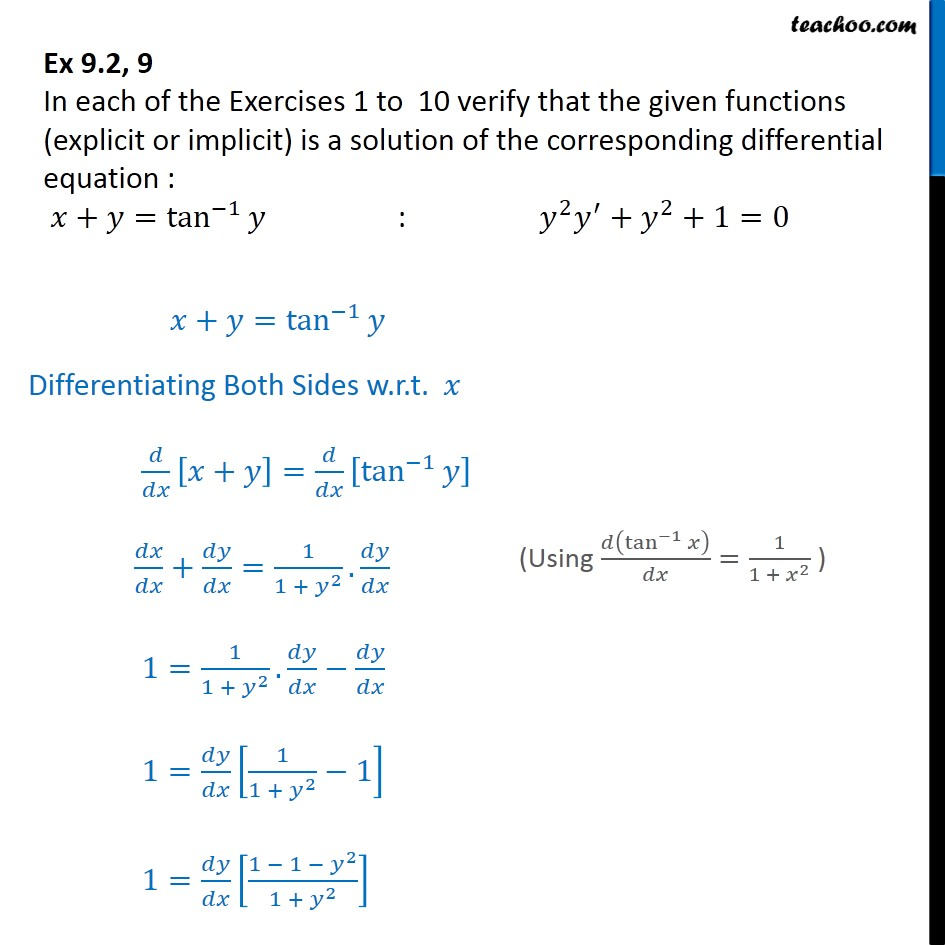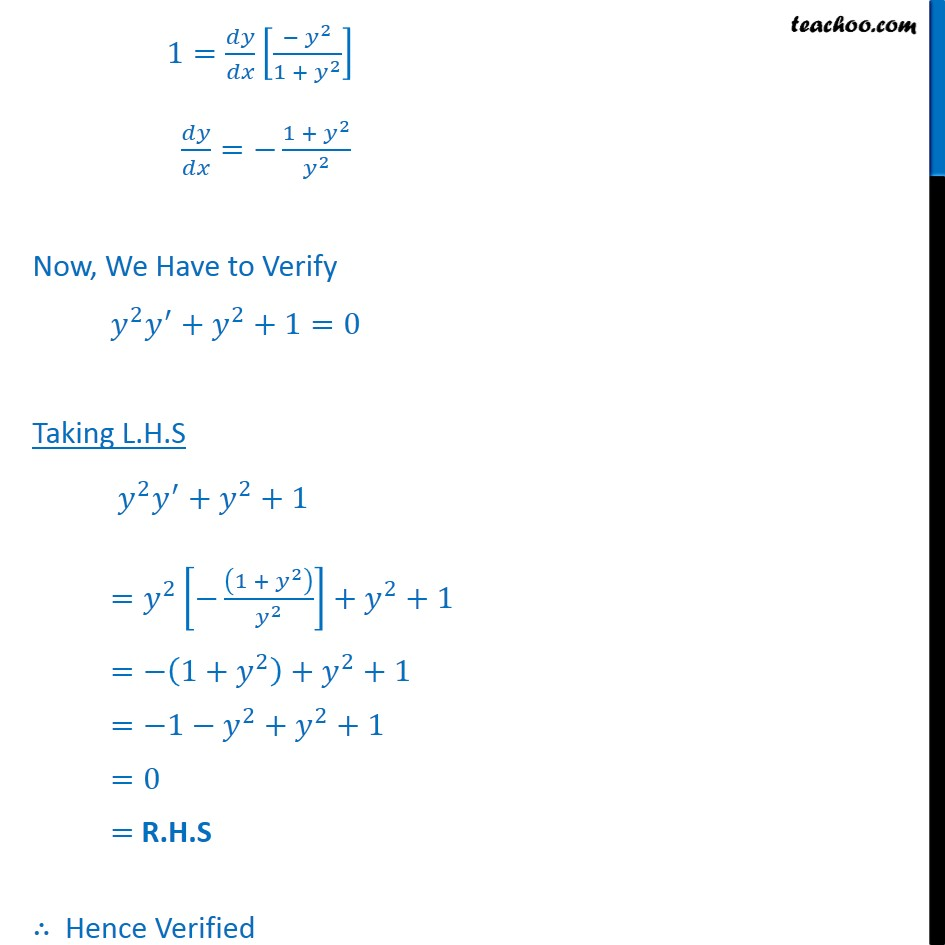Ex 9.2

Chapter 9 Class 12 Differential Equations
Serial order wiseLearn in your speed, with individual attention - Teachoo Maths 1-on-1 Class

### Transcript

Ex 9.2, 9 In each of the Exercises 1 to 10 verify that the given functions (explicit or implicit) is a solution of the corresponding differential equation : 𝑥+𝑦= tan﷮−1﷯﷮𝑦﷯ : 𝑦﷮2﷯ 𝑦﷮′﷯+ 𝑦﷮2﷯+1=0 𝑥+𝑦= tan﷮−1﷯﷮𝑦﷯ Differentiating Both Sides w.r.t. 𝑥 𝑑﷮𝑑𝑥﷯ 𝑥+𝑦﷯= 𝑑﷮𝑑𝑥﷯ tan﷮−1﷯﷮𝑦﷯﷯ 𝑑𝑥﷮𝑑𝑥﷯+ 𝑑𝑦﷮𝑑𝑥﷯= 1﷮1 + 𝑦﷮2﷯﷯. 𝑑𝑦﷮𝑑𝑥﷯ 1= 1﷮1 + 𝑦﷮2﷯﷯. 𝑑𝑦﷮𝑑𝑥﷯− 𝑑𝑦﷮𝑑𝑥﷯ 1= 𝑑𝑦﷮𝑑𝑥﷯ 1﷮1 + 𝑦﷮2﷯﷯−1﷯ 1= 𝑑𝑦﷮𝑑𝑥﷯ 1 − 1 − 𝑦﷮2﷯﷮1 + 𝑦﷮2﷯﷯﷯ 1= 𝑑𝑦﷮𝑑𝑥﷯ − 𝑦﷮2﷯﷮1 + 𝑦﷮2﷯﷯﷯ 𝑑𝑦﷮𝑑𝑥﷯=− 1 + 𝑦﷮2﷯﷮ 𝑦﷮2﷯﷯ Now, We Have to Verify 𝑦﷮2﷯ 𝑦﷮′﷯+ 𝑦﷮2﷯+1=0 Taking L.H.S 𝑦﷮2﷯ 𝑦﷮′﷯+ 𝑦﷮2﷯+1 = 𝑦﷮2﷯ − 1 + 𝑦﷮2﷯﷯﷮ 𝑦﷮2﷯﷯﷯+ 𝑦﷮2﷯+1 =− 1+ 𝑦﷮2﷯﷯+ 𝑦﷮2﷯+1 =−1− 𝑦﷮2﷯+ 𝑦﷮2﷯+1 =0 = R.H.S ∴ Hence Verified